## Introduction

In the information age, the Internet is becoming a de facto public good, akin to roads, airports or any other critical infrastructure1. According to the Internet World Stats, more than a thousand million people are estimated to use the Internet every day, to communicate, search for information, share data or do business. Online social networks are becoming an integral part of human social activities, increasingly affecting human psychology2. Underlying all these processes is the Internet infrastructure, composed, on a large scale, of connections between autonomous systems (ASs). An AS is, roughly, a part of the Internet owned and administered by the same organization3. ASs range in size from small companies, or even private users, to huge international corporations. No central Internet authority exists that dictates to any AS what other ASs to connect to. Connections between ASs are results of local independent decisions based on business agreements between AS pairs. This lack of centralized engineering control makes the Internet a truly self-organized system, and poses many scientific challenges. The one we address here is the sustainability of Internet growth.

The Internet has been growing fast according to all measures4,5. For example, the number of ASs increases by ~2,400 every year4. Despite its growth, the Internet must sustainably perform its primary task—routing information packets between any two computers in the world. But can this function be really sustained? To route information to a given destination in the Internet today, all ASs must collectively discover the best path to each possible destination, based on the current state of the global Internet topology. As the number of destinations grows quickly, the amount of information each AS has to maintain becomes a serious scalability concern, endangering the performance and stability of the Internet6. Worse yet, the Internet is not static. Its topology changes constantly because of the failure of existing links and nodes, or because of the appearance of new ones. Each time such a change occurs anywhere in the Internet, the information about this event must be diffused to all ASs, which have to quickly process it to recompute new best routes. The constantly increasing size and dynamics of the Internet thus leads to immense and quickly growing routing overheads, causing concerns among Internet experts that the existing Internet routing architecture may not sustain even another decade6,7,8,9; parts of the Internet have started sinking into black holes already10.

In our recent work11,12,13,14,15, we have shown that greedy forwarding is indeed efficient in Internet-like synthetic networks embedded in geometric spaces, and that this efficiency is maximized if the space is hyperbolic. However, putting these ideas in practice needs a crucial piece of information: a map of the real Internet in a hyperbolic space. Here, we present a method to find such a map. Our method uses statistical inference techniques to find coordinates for each AS in the hyperbolic space underlying the Internet. Guided by the inferred coordinates, greedy forwarding in the Internet achieves efficiency and robustness, similar to those in synthetic networks. We also find that the method maps geo-politically close ASs close to each other in the hyperbolic space. This finding suggests that our mapping method can be used for soft community detection in real networks, where by soft communities we mean groups of geometrically close nodes.

## Results

To build a geographical map, one first has to model the Earth surface, for example, by assuming that it is a sphere. Similarly, we also need a geometric model of the Internet space to build our map. The simplest candidate space is also a sphere, or even a circle, on which nodes are uniformly distributed and connected by an edge, with probability p(d) decreasing as a function of distance d between nodes, conceptually similar to random geometric graphs16. However, this model fails to capture basic properties of the Internet topology, including its scale-free node degree distribution. In an earlier study17, we showed that to generate realistic network topologies in this geometric approach, we first have to assign to nodes their expected degrees κ drawn from a power-law distribution, and then connect pairs of nodes with expected degrees κ and κ′ with probability p(χ), where χ is distance d rescaled by the product of the expected degrees, χ~–d/(κκ′). We thus have a hybrid model that mixes geometry and topology—geometric characteristics, distances d used in random geometric graphs, come in tandem with topological characteristics, expected degrees κ used in classical configuration models of random power-law graphs18. If we associate the expected degree κ of a node with its mass, then the connection probability p(d/(κκ′)), which is a measure of the interaction strength between two nodes, resembles Newton's law of gravitation. Therefore, we call this model Newtonian. However, according to Einstein, we can treat gravity in purely geometric terms if we accept that the space is no longer flat, that is, if it is non-Euclidean. Following this philosophy we showed in an earlier study13 that the Newtonian model is isomorphic to a purely geometric network model, with node degrees transformed into a geometric coordinate, making the space hyperbolic, that is, negatively curved. We call this model Einsteinian.

The main property of hyperbolic geometry is the exponential expansion of space illustrated in Figure 1. For example, the area A(r) of a two-dimensional hyperbolic disc of radius r grows with r as A(r)~er. Consequently, the uniform node density in a hyperbolic space appears as exponentially growing with the distance r from the origin (see Figure 2, illustrating the Einsteinian model). In the model, nodes are indeed distributed (quasi-)uniformly on a hyperbolic disc, and one can show13 that the resulting average degree of nodes exponentially decreases with r. This combination of two exponentials, node density and average degree, leads to the emergence of a scale-free degree distribution in the network. The model is described in the Methods section, and can generate synthetic scale-free networks with any power-law degree distribution exponent and any clustering. Given a real network, our network mapping method, also in the Methods section, reverts the network synthesis in the model. The method uses statistical inference techniques to identify the hyperbolic coordinates for each node in the given network, which would maximize the likelihood that the network is generated by the model. Specifically, the method attempts to find node positions such that the resulting empirical probability of node connections as a function of the hyperbolic distance between nodes would be congruent with the theoretical connection probability in the model.

### Mapping results

We apply our mapping method to the Internet AS topology extracted from the Archipelago project data19 in June 2009, and visualize the results in Figure 3. We observe striking similarity between this visualization and the synthetic Einsteinian network in Figure 2. To confirm that the Internet map we have obtained is indeed congruent with the Einsteinian model, we juxtapose in Figure 4 the empirical connection probability between ASs in the obtained Internet map against the theoretical one in Equation (4) of the Methods section. We observe a clear similarity between the two. Neither is the sphere a perfect model of the Earth nor is the Einsteinian model an ideal abstraction of the Internet structure. Yet, the observed similarity between the empirical and theoretical connection probabilities in Figure 4 suggests that hyperbolic metric spaces are reasonable representations of the real Internet space.

To investigate further the connections between the obtained map and Internet reality, we show in Figure 3 the average angular position of all ASs belonging to the same country, whereas in Figure 5 we draw the angular distributions of those ASs. Surprisingly, we find that even though our mapping method is completely geography agnostic, it discovers meaningful groups or communities of ASs belonging to the same country. Furthermore, in Figure 3, we find many cases of geographically or politically close countries placed close to each other in our hyperbolic map. The explanation of these surprising effects is rooted in the peculiar nature of our mapping method. If ASs belonging to the same country, geographic region or geo-political or economic group are connected more densely to each other than to the rest of the world, then this higher connection density translates to a higher attractive force that tries to place all such ASs close to each other in our map. Indeed, the term $p{\left({x}_{ij}\right)}^{{a}_{ij}}$ in Equation (7) of the Methods section corresponds to the attractive force between connected nodes, whereas the term ${\left[1-p\left({x}_{ij}\right)\right]}^{1-{a}_{ij}}$ is the repulsive force between disconnected ones. This peculiar interplay between attraction within densely connected regions and repulsion across sparsely connected zones effectively maps the ASs belonging to densely connected AS groups closely. These observations build our confidence that our mapping method provides meaningful results reflecting peculiarities of the real Internet structure, and suggest that the method can be adapted to discover the community structure20,21,22 in other complex networks.Figure 5: Angular positions of ASs belonging to the same country.

### Routing results

The obtained Internet map is ready for greedy forwarding. An AS holding a packet reads its destination AS coordinates, computes the hyperbolic distances between this destination and each of its AS neighbours using Equation (3) of the Methods section and forwards the packet to the neighbour closest to the destination. To evaluate the performance of this process, we perform greedy forwarding from each source to each destination AS, and compute several performance metrics.

The first metric is success ratio, which is the percentage of greedy paths that successfully reach their destinations. Not all paths are expected to be successful, as some might run into local minima. For example, an AS might forward a packet to its neighbour who sends the packet back to the same AS, in which case the packet will never reach the destination. We declare a path unsuccessful if the packet is sent to the same AS twice. The average success ratio of simple greedy forwarding in our Internet map is remarkably high, 97%, and more sophisticated greedy forwarding techniques, such as those described in Cvetkovski and Crovella study23, can boost it to 100%. Given the discussed connections between our Internet map and geography, one may conjecture that greedy forwarding simply mimics geographical routing following the geographically shortest paths. However, this conjecture is not true. Geography is reflected in our map only along the angular coordinate, whereas the radial coordinate is a function of the AS degree, making the space hyperbolic (see the Methods section). The geographical space is not hyperbolic, and if we use it for greedy forwarding, we obtain a much lower success ratio of approximately 14%. We also tested modified geographic routing that tries to intelligently use AS degrees, in the spirit of our Einsteinian model. Nevertheless, this modification, although improving the success ratio to 30%, still falls short compared with the results obtained using our hyperbolic map. The details of these experiments with geographical routing can be found in Supplementary Methods.

The second metric is stretch, which tells us how much longer the greedy paths are compared with the shortest paths in the Internet topology. The average stretch is low, 1.1. The average hop-wise length of the shortest paths between selected sources and destinations is 3.49, so that the average length of greedy paths is 3.86. The low value of stretch indicates that greedy paths are close to optimal, that is, they are the shortest paths. The shortest path between nodes a and b in Figure 2, for example, is also the path found by greedy forwarding. Somewhat unexpectedly, the greedy stretch is asymptotically optimal, that is, equal to 1, in scale-free, strongly clustered networks, regardless of what underlying space is used for greedy forwarding12. Low stretch also implies that greedy forwarding causes approximately the same traffic load on nodes as shortest-path forwarding. Given that shortest-path forwarding does not lead to high traffic load in scale-free networks24, this finding allays concerns that hyperbolic forwarding may cause traffic congestion abnormalities25 (see Supplementary Methods).

The two metrics above characterize the performance of greedy forwarding in the static Internet topology. More important than that is how greedy forwarding performs in the dynamic topology, in which links and nodes can fail. We randomly select a percentage of links and nodes, remove them from the mapped Internet, recompute the success ratio and stretch after the removal and finally present the result in the top plots of Figure 6. Even on simultaneous failures of up to 10% of AS links or nodes—catastrophic events never happened in Internet history—we observe only minor de-gradation of the performance of greedy forwarding. That is, even catastrophic levels of damage to the Internet do not significantly affect the performance of greedy forwarding, even though no AS changes its position on the hyperbolic map. A widely popularized feature of complex networks is their robustness with respect to random failures, and the lethality of failures of highest-degree hubs26,27. As expected, we observe in the bottom plots of Figure 6 that removals of such hubs have a more detrimental effect on greedy forwarding as well. However, targeted removal of highest-degree ASs in the Internet is a rather unrealistic scenario, as these large ASs consist of thousands of routers the simultaneous failure of which is a very rare and unlikely event. The explanation for the surprising efficiency of greedy forwarding with respect to random failures lies in the unique combination of the following two properties exhibited by scale-free, strongly clustered networks: high path diversity24, and congruency between hyperbolic geodesics and topologically shortest paths13,15. The latter is illustrated by the similar path patterns of the hyperbolic geodesic and topologically shortest path between nodes a and b in Figure 2: they both first go to the high-degree core of the network, and then exit it in the appropriate direction to the destination. Owing to high path diversity, there are many disjoint shortest paths between the same source and destination, and thanks to the congruency, they all stay close to the corresponding hyperbolic geodesics. Link and node failures affect some shortest paths, but others remain, and greedy forwarding can still find them using the same hyperbolic map.

Another form of Internet dynamics is its rapid growth over years4,5,28,29. We map the Internet of January 2007 to its hyperbolic space using the same mapping method, and then replay the historical growth of the Internet up to June 2009 with an interval of 3 months. During this two and a half year replay, we keep the AS coordinates, as soon as they are computed, fixed once and forever, whereas the ASs joining the Internet anew, after June 2007, compute their coordinates using a variation of the mapping method that requires only local topological information (see Supplementary Methods). In Figure 7a, we show the performance of greedy forwarding in the resulting maps at each time step, and observe only minor performance degradation, even over long time scales. In a nutshell, the existing AS coordinates are essentially static, as once computed they can stay the same for years.Figure 7: Performance of greedy forwarding during the replayed historical growth of the Internet (a), and success ratio as a function of the fraction of missing links (b).

## Discussion

We have constructed a hyperbolic map of the Internet, and release this map as part of the Supplementary Data set. The map can be used for essentially infinitely scalable Internet routing. The amount of routing information that ASs must maintain is proportional to the AS degree, which is theoretically best possible as ASs must always keep some information about their neighbours. Routing communication overheads are also minimized, as ASs do not exchange any routing information on dynamic changes of the AS topology. The presented solution thus achieves routing efficiency that is theoretically close to optimal, and resolves serious scaling limitations that the Internet faces today.

The mapping method we have used is generic, and can be applied to other complex networks with underlying metric structures and heterogeneous degree distributions. We showed in an earlier study17 that a good indicator for the presence of an underlying metric structure is self-similarity of clustering in the network, whereas in an earlier study13 we showed that as soon as a metric space is present, and the network has a heterogeneous degree distribution, the metric distances can be rescaled such that the underlying geometry is effectively hyperbolic. Roughly, self-similar clustering is responsible for the metric structure along the angular coordinate, whereas degree heterogeneity adds the radial dimension and makes the space hyperbolic. Applied to other networks, our mapping method can provide a different perspective on the community structure in networks. Instead of trying to split nodes into discrete community sets20,21,22, it would naturally yield a continuous measure of similarity between nodes on the basis of hyperbolic distances. More similar nodes would be located closer to each other, and form zones of higher connectivity density. Thereafter, it would be up to an experimenter to define communities, if needed, as histograms of the node density in the hyperbolic space. The spectrum of potential applications of this network-mapping geometrization agenda is wide. Network mapping can reveal geometric forces effectively driving information signalling in the network; examples include the brain30 and cell signalling networks31. One can then potentially predict what network perturbations drive these networks to failure, such as brain disorders or cancer. Other applications range from recommender systems32, in which the right measure of similarity between consumers is a key, to epidemic spreading33 and information theory of networks34.

We have shown that the Internet hyperbolic map is remarkably robust with respect to even substantial perturbations of the Internet topology, implying that this map is essentially static. It does not significantly depend on topology dynamics, and can thus be computed only once. This property is desirable in view of long running times intrinsic to likelihood maximization algorithms. Our method improves their running times drastically, and the Internet map computations take approximately a day on a modern computer. However, for substantially larger networks, the running times may still be prohibitive even for one-time mapping. Therefore, alternative methods for network mapping, not relying on likelihood maximization, are highly desirable, and our work in this direction is underway.

## Methods

### The Einsteinian and Newtonian models of complex networks

To synthesize a network with our Einsteinian model, one has to first specify any desired network size N, as well as average degree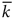, average clustering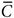and exponent γ>2 of the power-law distribution P(k) of node degrees k, P(k)~kγ. Equipped with these target properties of the network topology, we first distribute N nodes (quasi-)uniformly within a hyperbolic disc of radius R=2log(N/c), where c is given by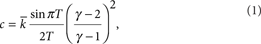and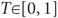is a function of. In the hyperbolic plane, the quasi-uniform node density means that the node angular coordinates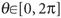are distributed uniformly, whereas their radial coordinates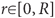are distributed with density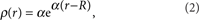where α=(γ−1)/2. Once all nodes are in place, specified by their assigned coordinates, the hyperbolic distance xij between each pair of nodes i and j located at (ri,θi) and (rj,θj) is computed using the hyperbolic law of cosines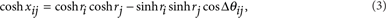where Δθij is the angle between segments connecting the origin and points i and j. On distributing nodes over the disc as described, we form scale-free networks in the model by connecting each pair of nodes i and j located at hyperbolic distance xij with the connection probability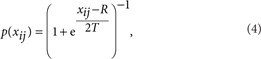almost identical to the Fermi-Dirac distribution in statistical mechanics. It depends only on hyperbolic distances xij (link energies), the hyperbolic disc radius R (chemical potential) and parameter T≥0 (temperature) controlling network clustering. After each node pair is examined and connected with probability p(xij), the network is formed and we can compute the average degree k(r) of nodes located at distance r from the origin. The result is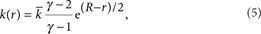which, combined with Equation (2), yields the target degree distribution P(k). The Newtonian model is isomorphic to the Einsteinian one through a simple change of variables reminiscent of Equation (5):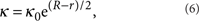where κ is the expected degree of a node in the Newtonian model, and κ0 is the minimum expected degree. See Krioukov et al.13 for further details.

### The mapping method

As our goal is to build a realistic Internet map, ready for routing and other applications, we have to find for each AS its radial and angular coordinates (r,θ), maximizing the efficiency of greedy forwarding. This specific task of maximizing greedy forwarding efficiency calls for a mapping method different from existing techniques on embedding Internet distances and graphs35,36,37. In view of our previous findings11,12,13,14,15 that greedy forwarding is exceptionally efficient in Internet-resembling synthetic networks, and that this efficiency is maximized in the Einsteinian model, our strategy for the Internet map construction is to maximize the congruency between the map and the model. In statistical inference38, this goal is equivalent to maximizing the likelihood that the observed data, that is, the Internet topology, has been produced by the model. This likelihood is given by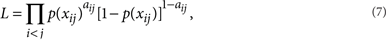where the elements aij of the Internet adjacency matrix are equal to 1 whenever there exists a connection between ASs i and j, and to 0 otherwise. Whereas the adjacency matrix represents the observed data, the connection probability p(xij) depends, by means of Equations (4, 3), on the AS coordinates (r,θ), which we try to infer. Our best estimate for these coordinates is then those maximizing the likelihood in Equation (7).

Although there are plenty of methods to find maximum-likelihood solutions, for example, the Metropolis–Hastings algorithm39, they perform poorly and do not scale well on large data sets with abundant local maxima, which is the case with the Internet. Therefore, as important as a likelihood maximization method is a heuristic approach helping the maximization algorithm to find the optimal solution in a reasonable amount of time and with reasonable computational resources. Our method is based on the following remarkable property of networks in our model; the same property holds for the Internet17. Let G be a given network with average degreeand power-law degree distribution P(k)~kγ, and let G(kT) be G's subgraph composed of nodes with degree larger than some threshold kT, along with the connections among these nodes. The average degree in G(kT) is then given by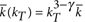.17 In scale-free networks with exponent γ between 2 and 3, this internal average degree is thus a growing function of kT, which implies that subgraphs made of high-degree nodes almost surely form a single connected component. Using this property, along with the statistical independence of the graph edges, it becomes possible to infer coordinates of ASs in G(kT) ignoring the remainder of the AS graph. This property is practically important because the size of G(kT) decreases very fast as kT increases, which speeds up likelihood maximization algorithms tremendously. In a nutshell, our method starts with a subgraph G(kT) small enough for standard maximization algorithms being able to reliably and quickly infer the coordinates of ASs in G(kT). Once these are found, we gradually increase kT to iteratively add layers of lower-degree ASs. While doing so, we use the already inferred AS coordinates as a reference frame to assign initial coordinates to newly added ASs. This initial coordinate assignment significantly improves the convergence time of maximization algorithms. All other details of our mapping method can be found in Supplementary Methods.

### The archipelago Internet topology

We use the AS Internet topology of June 2009 extracted from data collected by the archipelago active measurement infrastructure developed by Cooperative Association for Internet Data Analysis19. The AS topology contains 23752 ASs and 58416 AS links, yielding the average AS degree= 4.92 . The maximum AS degree is kmax=2778. The average clustering measured over ASs of degree larger than 1 is= 0.61, yielding temperature T=0.69, and hyperbolic disc radius R=27. The exponent of the power-law AS degree distribution is γ=2.1. This Internet topology is available as part of the Supplementary Data set, along with the hyperbolic Internet map.